Next: Steered Molecular Dynamics Up: Analysis Previous: Equilibrium

Subsections

## Non-equilibrium properties of protein

This section contains two exercises that present situations in which the ubiquitin system is not at equilibrium. This section requires some knowledge of the principles of statistics physics, but the results of the simulations can be understood intuitively even without a deep understanding of their principles.

### Heat Diffusion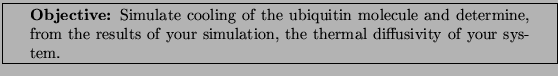For this exercise, you will use the last step of the equilibration of ubiquitin in a water sphere ubq_ws_eq.restart.coor. You will use the temperature coupling feature of NAMD to set the temperature of the molecules in the outer layer of the sphere to 200 K. You will determine the thermal diffusivity by monitoring changes of the system's temperature and comparing them to the theoretical expression.* 1
Change to this problem's directory:

 cd 2-6-heat_diff/

In order to use the temperature coupling feature of NAMD2, you need to create a file which marks the atoms subject to the temperature coupling. The following lines
tCouple on
tCoupleTemp 200
tCoupleFile ubq_shell.pdb
tCoupleCol B
in the provided configuration file ubq_cooling.conf will enable this feature, and set the atoms coupled to the thermal bath as the ones that have a value of 1.00 in the B column of the ubq_shell.pdb

2
Launch VMD.

3
Open the VMD TkCon window by choosing the Extensions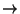tkcon menu item.

4
Load the system into VMD by typing the following in the VMD tkcon window:

 mol load psf ubq_ws.psf namdbin ubq_ws_eq.restart.coor

5
Select all atoms in the system:
set selALL [atomselect top all]

6
Find the center of the system:
set center [measure center $selALL weight mass] 7 Find,andcoondinates of the system's center: foreach {xmass ymass zmass}$center { break }
8
Select atoms in the outer layer:
set shellSel [atomselect top "not ( sqr(x-$xmass) + sqr(y-$ymass) + sqr(z-$zmass) <= sqr(22) ) "] 9 Set beta parameters of the atoms in this selection to 1.00:$shellSel set beta 1.00

10
Select the entire system again:
set selALL [atomselect top all]

11
Create the pdb file that marks the atoms in the outer layer by 1.00'' in the beta column:
$selALL writepdb ubq_shell.pdb 12 You can now quit VMD.* 13 Use namdplot to find out how the system's temperature changes with time: namdplot TEMP ubq_cooling.log The xmgrace program window will pop up.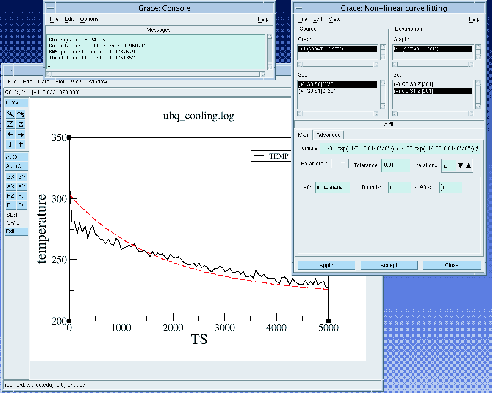* 14 In the xmgrace program, choose the DataTransformationsGeometrical transformmenu item. In the Scale X: window, type 2.0, which corresponds to the time step used in your simulation. Press Accept, which will rescale the plot such that the-axis is now in units of fs. * 15 You will now fit the simulated temperature dependence to the theoretical expression (see the Science Box). For this, you will use the Non-linear curve fitting feature of xmgrace. * 16 Choose the DataTransformationsNon-linear curve fitting... menu item. In the SourceSet window, click on the last line, which corresponds to the simulated time dependence. * 17 In the Main tab, type or copy and paste over the Formula window (make sure it is a one line): y= 200 + 66.87*(exp( -0.0146*a0*x) +0.25*exp( -0.25*0.0146*a0*x) +1/9*exp(-1/9*0.0146*a0*x) +1/16*exp(-1/16*0.0146*a0*x) +1/25*exp(-1/25*0.0146*a0*x) +1/36*exp(-1/36*0.0146*a0*x) +1/49*exp(-1/49*0.0146*a0*x) +1/64*exp(-1/64*0.0146*a0*x) +1/81*exp(-1/81*0.0146*a0*x) +1/100*exp(-1/100*0.0146*a0*x) ) * 18 In the Parameters drop-down menu, choose 1. Windows will appear below. Click several times on Apply, to get a better fit. This will fit the curve and get a value for the parameter a0, which is the thermal diffusivity. * 19 Multiply the value of theparameter by 0.1 to get the thermal diffusivity in cms. You should get a value of around 0.45cms. How does your result compare to the thermal diffusivity of water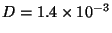cms? 20 Compute the thermal conductivity of the system,, by the following formula: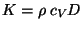, whereis the specific heat (that was calculated in Section 2.1.5) andis the thermal diffusivity (assume that the density of the system,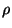, is 1 g/mL). ### Temperature echoesThe motions of atoms in globular proteins (e.g., ubiquitin), referred to as internal dynamics, comprise a wide range of time scales, from high frequency vibrations about their equilibrium positions with periods of several femtoseconds to slow collective motions which require seconds or more, leading to deformations of the entire protein. The internal dynamics of these proteins on a picosecond time scale (high frequency) can be described as a collection of weakly interacting harmonic oscillators referred to as normal modes. Since normal modes are formed by linear superposition of a large number of individual atomic oscillations, it is not surprising that the internal dynamics of proteins on this time scale has a delocalized character throughout the protein. The situation is similar to the lattice vibrations (phonons) in a crystalline solid. Experimentally, there exist ways to synchronize, through a suitable signal or perturbation, these normal modes, forcing the system in a so-called (phase) coherent state, in which normal modes oscillate in phase. The degree of coherence of the system can be probed with a second signal which through interference with the coherent normal modes may lead to resonances, referred to as echoes, which can be detected experimentally. However, the coherence of atomic motions in proteins decay through non-linear contributions to forces between atoms. The decay of coherence develops on a time scale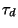which can be probed, e.g., by means of temperature echoes, and can be described by employing MD simulations. In a temperature echo the coherence of the system is probed by reassigning the same atomic velocities the system had at an earlier time and then looking to an echo in the temperature at time, as a result of such reassignment. An example is shown in Fig.13. At time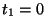the velocities of all atoms in the system are quenched; then, at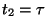the atomic velocities are reassigning again (quenched) to their values at time. As a result, a temperature echo, i.e., a sharp dip in, is detected at a subsequent time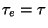after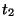(i.e., at time). In this section, you will employ MD simulations to generate temperature echoes in ubiquitin by applying the velocity reassignment just described. By modeling ubiquitin as a large collection of weakly interacting harmonic oscillators (normal modes), you will find that: • the dephasing timeof the oscillators is about one picosecond; • the temperature echo can be expressed in terms of the temperature autocorrelation function; and • the depthof the echoes decay exponentially with the delay time, i.e.,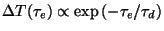, the exponential decay being determined by the dephasing time.First you will generate temperature echoes in MD simulations, and then you will analyze the echoes in the framework of the normal mode approximation.The phenomenon of temperature echoes has a simple explanation within the normal modes description of a protein. The first velocity reassignment enforces phase coherence for the oscillators. Because of the frequency dispersion of the normal modes (as well as, the deviation from the harmonic approximation) the phase coherence of the protein will decay in time with a characteristic dephasing time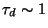ps. The second velocity reassignment after a delay timeis a probing signal'' which will test the degree of coherence of the system at the instant of time it was applied. The depth of the echo and the instant of time at which it occurs are quantitative characteristics of the coherence of the internal dynamics of proteins. In order to generate temperature echoes, one needs to equilibrate ubiquitin at the desired initial temperature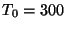K, e.g., by using the velocity rescaling method. Once the system is equilibrated, the thermostat is removed and all the following simulations are carried out in the microcanonical (NEV) ensemble. In this problem, you will consider the simplest temperature echo: the set of velocities of the second reassignment is exactly the same as the first one, i.e., without scaling. ### Temperature quench echo The temperature quench echo is obtained by resetting to zero the velocity of all atoms of the protein at both timesand. You should start from the pre-equilibrated protein in vacuum at; the required files are located in the common directory. You need to run the simulations in the microcanonical ensemble (NVE) by using the configuration file equil.conf in the 2-7-echoes/01_equil_NVE directory. The simulation will run for 500 time steps (fs). Let's take a look at some features in the configuration file: • The simulation takes restart files, i.e., the coordinate file and the velocity file, from an equilibration simulation at. # Continuing a job from the restart files set inputname ../common/ubi_equil binCoordinates$inputname.restart.coor
binvelocities $inputname.restart.vel • Notice that the temperature line is commented out. The initial temperature is pre-defined by the initial velocities. #temperature$temperature

• Since you are running an NVE simulation, there is no temperature coupling or pressure control.

• The time step is 1fs, and the rigidBonds option is turned off.1
Once your job is done, you need to get the temperature data from the output file. Do this by using the script gettemp:
gettemp equil.log > temp1.dat
This will write the data into the file temp1.dat.

You will now calculate the temperature autocorrelation function, which will be used later to analyze the temperature echoes.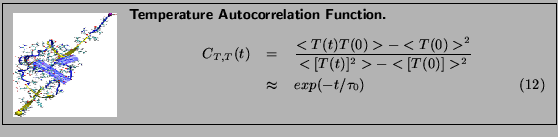2
Start Matlab, by typing in a terminal:
tbss> matlab -nosplash &

3
In the Command Window, type:
» data_analysis

This will calculate the temperature autocorrelation function and put it in the file auto_tmp.dat.

A plot of the autocorrelation function will appear on your screen (c.f. Fig. 14) in a dotted curve. The solid curve is a fit to an exponential function of Eq. 12. The decay (temperature autocorrelation) timefrom this exponential is calculated and displayed in the Command Window. This value will be important for a later analysis of your simulations. The value is stored in the file tau0.dat.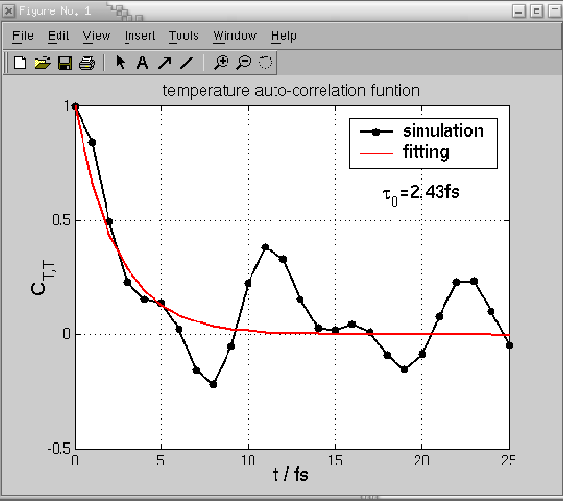4
Go to the next directory: 02_quencha by typing in a terminal:
cd ../02_quencha

5
You will make the temperature quenching experiment for a particularthat will be assigned to you. Go to
http://www.ks.uiuc.edu/deyulu/thermal_echo.html
to find out which value you should use.

Once you know your value for, you are going to quench the temperature (set it to zero), and monitor the recovery of the system as it runs fortime steps.

6
Open your configuration file by typing in a terminal:
nedit echo.conf

7
Change the value of tau to the value you were assigned:
set tau 200 ;# here you want to assign your tau value
Save and close the file.8
Get the temperature data from the output file of the simulation by typing:
gettemp echo.log > temp2.dat
This will write the data into the file temp2.dat.

Now, you will quench the system for the second time.

9
Change to the directory 03_quenchb/

10
You need to modify the configuration file echo.conf with the appropriate value ofagain. Note that this time the simulation will run forsteps:
run [expr 3*$tau]11 Get the temperature data: gettemp echo.log > temp3.dat This will write the data into the file temp3.dat. 12 Copy the files temp1.dat and temp2.dat that you generated before to this directory: cp ../01_equil_NVE/temp1.dat . cp ../02_quencha/temp2.dat . 13 Merge the three temperature data files into temp.dat: cat temp[1-3].dat > temp.dat You now have the temperature data for the whole temperature quench experiment. You will use Matlab to analyze the data. 14 Start Matlab as before by typing: matlab -nosplash & in a terminal. 15 Load the file temp.dat, by typing in the Matlab Command Window: » load temp.dat 16 Plot the temperature data by typing: » plot(temp(:,1),temp(:,2)) This command will plot the time in the x axis and the temperature in the y axis (Fig. 15 (A)). Note anything unusual? You quenched the temperature twice, and yet you see three drops in temperature! The third one is a temperature echo.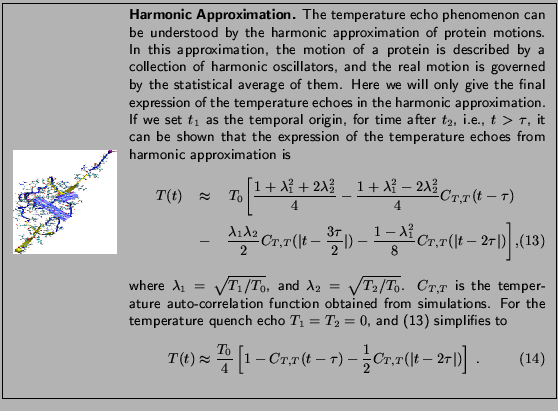Next, you will comparein the vicinity of the echo () obtained from the MD simulation with the theoretical prediction (14) involving the temperature autocorrelation function obtained also from the MD simulations. The degree of agreement between these two results is a measure of the accuracy of the harmonic approximation. 17 You will use a Matlab script that defines a function called cmp_echo. This will plot both the simulatedand the one given by Eq. 14 (Fig. 15 (B)). Use this function by typing: » cmp_echo(tau,temp) where tau is the delay time tau assigned to you. You may also want to use the zoom buttons on the tools menu of the Matlab figure window to magnify the details of the echo. 18 You need to find the depth of the echo. Do this by looking at the plot, or by typing the command: » 75 - min(temp(510+tau:end,2)) where 75 is the average temperature after the second quench, and it corresponds to 1/4 of the initial temperature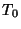=300K. Note that this formula will not catch the echo for larger values of tau (e.g. tau=800 fs). 19 Each of you will calculate the echo depth for a particular value of the delay time. You need to input the echo depth at http://www.ks.uiuc.edu/deyulu/thermal_echo.html, and you will later see a plot showing the data collected from all of you. By fitting them to an single exponentialone obtains the so called dephasing time, which is an inherent property of the system.### OPTIONAL EXERCISE: Velocity replacement echo Here you will generate a temperature echo by replacing the velocities of the atoms at timewith the ones you assign to them at time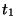. Moreover, by choosing these velocities according to the Maxwell-Boltzmann distribution corresponding toK, i.e., the temperature of the equilibrated system (), there will be no discontinuities (jumps) inatand, yet you will observe a temperature echo at a later time, i.e., a sharp dip in the temperature at time. Your goal is to determineand the depthof the echo. To this end, you will need to follow a similar procedure to the one in the previous exercise. 20 Go to the directory 2-7-echoes/04_consta/. Atyou should reassign the velocities according to a Maxwell-Boltzmann distribution for a temperature. Make sure that you save the reassigned velocities to a file 300.vel. 21 In order to do this, the following is added to the end of your configuration file echo.conf:  run 0 output 300 This settings will not really run a simulation, but will assign initial velocities with the desired distribution and save them to 300.vel. Following these, the normal run command is used to perform a simulation for tau time steps:  run$tau22
Get the temperature data as before and put it in the file temp2.dat. Note that at the beginning there is a duplicate entry for the first time step. You should delete one of these:
500 300.5656
500 300.5656
501 301.0253
502 302.5395
...
23
Go to the directory 05_constb/.
24
For this simulation, you should use the file 300.vel you generated before as the velocity restart file. This is included in the configuration file echo.conf as:
velocities ../04_consta/300.vel
This will reassign the velocities to the exact same distribution they had at the beginning of the previous simulation. This simulation will run for 3time steps.25
Again get the temperature data and put it in the file temp3.dat.
26
Copy the files temp1.dat and temp2.dat into this directory:
cp ../01_equil_NVE/temp1.dat .
cp ../04_consta/temp2.dat .
Merge the three temperature data files into temp.dat.
27
Start Matlab and use the script cmp_echo.m to analyze your data.
28
Try to locate the position of the echo. This time, the depth of the echo is measured by:
» 300 - min(temp(510+tau:end,2))
29
Compare your result with the pre-made results stored in the directory example_data for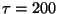fs. How would you explain your findings ?Next: Steered Molecular Dynamics Up: Analysis Previous: Equilibrium
namd@ks.uiuc.edu